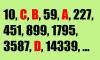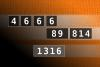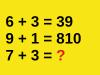BRAIN TEASERSBrain Teasers User Profile

# Stefan Stankovic

rank
421
points
5
See full ranking list
short ranking list
 419 sajed mohamadi asl 5 420 fio violeta 5 421 Stefan Stankovic 5 422 Наталья Мирра 5 423 CU29 Uroš 5
 Calculate A+B+C+D Look at the series (10, C, B, 59, A, 227, 451, 899, 1795, 3587, D, 14339, ...), determine the pattern, and find the unknown values (A, B, C and D) and calculate A+B+C+D!Calculate the number 1316 NUMBERMANIA: Calculate the number 1316 using numbers [4, 6, 6, 6, 89, 814] and basic arithmetic operations (+, -, *, /). Each of the numbers can be used only once.Mathematical Puzzle: IF 6+3=... Mathematical Puzzle: IF 6+3=39, 9+1=810 and 6+5=111 THEN 7+3=?